Ohm's law and resistance

# Aim:

To determine the resistance per cm of a given wire by plotting a graph of potential difference versus current, and hence to determine its resistivity.

# The Theory:

What does Ohm's Law state?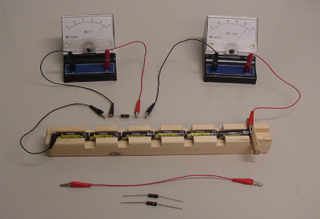According to the Ohm's law, "The current flowing through a conductor is directly proportional to the potential difference across its ends provided the physical conditions (temperature, dimensions, pressure) of the conductor remains the same." If I be the current flowing through a conductor and V be the potential difference across its ends, then according to Ohm's Law,

##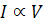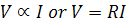where, R is the constant of proportionality. It is known as resistance of the conductor. Or

##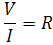..the working formula

R depends upon the material, temperature and dimensions of the conductor.

In S.I. units, the potential difference V is measured in volt and the current I in ampere, the resistance R is measured in ohm.

How do we establish the current-voltage relationship?

To establish the current-voltage relationship, it is to be shown that the ratio V / I remains constant for a given resistance, therefore a graph between the potential difference(V) and the current (I) must be a straight line.

How then do we find the unknown values of resistance?

It is the constant ratio that gives the unknown values of resistance,

##For a wire of uniform cross-section, the resistance depends on the length l and the area of cross-section A. It also depends on the temperature of the conductor. At a given temperature the resistance,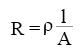where ρ is the specific resistance or resistivity and is characteristic of the material of wire.

Hence, the specific resistance or resistivity of the material of the wire,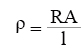If 'r' is the radius of the wire, then the cross sectional area, A = πr². Then the specific resistance or resistivity of the material of the wire is,# Learning Outcomes:

• Students learn Ohm’s law.
• Students know the relation between voltage, current and resistance.
• Students learn how to find out the resistivity of the material  of a give wire.